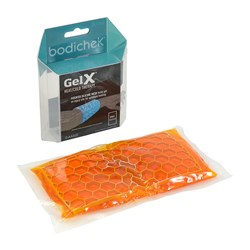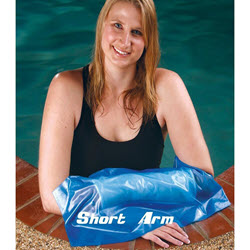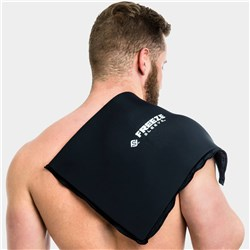# Rehabilitation

Grid List
\$21.10
Increase value Decrease value
\$100.87
Increase value Decrease value
\$100.87
Increase value Decrease value
\$100.87
Increase value Decrease value
\$230.00
Increase value Decrease value\$12.99
Increase value Decrease value
\$19.95
Increase value Decrease value
\$14.95
Increase value Decrease value
\$55.05
Increase value Decrease value
\$349.00
Increase value Decrease value\$33.00
Increase value Decrease value
\$59.00
Increase value Decrease value
Active Seal Adult Short Leg Cast Protector
\$59.00
Increase value Decrease value
Active Seal Adult Long Leg Cast Protector
\$59.00
Increase value Decrease value
\$59.00
Increase value Decrease value
\$713.90
Increase value Decrease value
P.O.A.
\$1452.00
Increase value Decrease value
\$7.22
Increase value Decrease value
\$6.49
Increase value Decrease value
\$27.94
Increase value Decrease value
\$19.57
Increase value Decrease value
\$7.33
Increase value Decrease value
\$169.95
Increase value Decrease value
\$62.05
Increase value Decrease value
Pquip Handy Grip Reacher 75cm (Pick Up)
\$49.28
Increase value Decrease value\$99.95
Increase value Decrease value
\$406.85
Increase value Decrease value
\$29.99
Increase value Decrease value
\$19.99
Increase value Decrease value
\$59.99
Increase value Decrease value
\$29.99
Increase value Decrease value
\$39.99
Increase value Decrease value
\$19.99
Increase value Decrease value
\$50.00
Increase value Decrease value
\$59.99
Increase value Decrease value
PTP Spinal Arch
In Stock
\$99.99
Increase value Decrease value
\$50.00
Increase value Decrease value
\$39.99
Increase value Decrease value
\$69.99
Increase value Decrease value
\$14.95
Increase value Decrease value
\$39.95
Increase value Decrease value
\$34.95
Increase value Decrease value
\$14.95
Increase value Decrease value
ObusForme High Back Support
\$262.49
Increase value Decrease value
\$23.40
Increase value Decrease value
\$46.33
Increase value Decrease value
BakBalls Regular
In Stock
\$39.95
Increase value Decrease value
Grid List
##### Products to compare:
Comparing Products